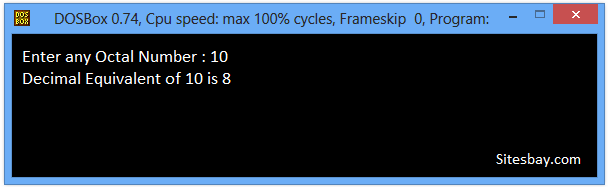# Convert Octal to Decimal Program in C++

## C++ program to Convert Octal to Decimal

To convert octal number to decimal number in C++ programming, we have to ask to the user to enter the octal number to convert it into decimal number to display the equivalent value in decimal format on screen.

## Example

```Input Octal Number : 26
Euivalent Decimal Number : 22

Input Octal Number : 10
Euivalent Decimal Number : 8

Input Octal Number : 15
Euivalent Decimal Number : 13
```

## C++ program to convert Octal to Decimal

```#include<iostream.h>
#include<conio.h>
#include<math.h>

void main()
{
int num, temp, rem, oct = 0, i = 0;
clrscr();
cout<<"Enter any Octal Number: ";
cin>>num;
temp = num;
while(temp != 0)
{
rem = temp % 10;
oct += rem * pow(8, i++);
temp = temp / 10;
}
cout<<"\nDecimal Equivalent of " << num << " is : " << oct;
getch();
}
```

## Output

```Enter any Octal Number : 10
Decimal Equivalent of 10 is 8
```## Pure VPN Privide Lowest Price VPN Just @ \$1.65. Per Month with Non Detected IP Lowest Price Non Detected IP VPN